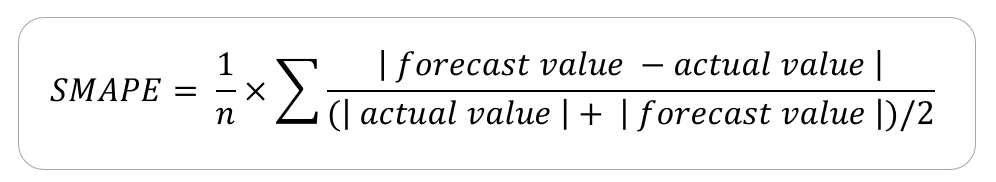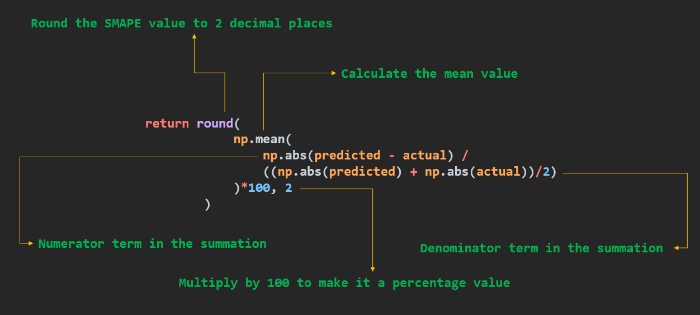Open in App
Not now

# How to Calculate SMAPE in Python?

• Last Updated : 28 Nov, 2021

In this article, we will see how to compute one of the methods to determine forecast accuracy called the Symmetric Mean Absolute Percentage Error (or simply SMAPE) in Python.

The SMAPE is one of the alternatives to overcome the limitations with MAPE forecast error measurement. In contrast to the mean absolute percentage error, SMAPE has both a lower bound and an upper bound, therefore, it is known as symmetric. The ‘S’ in SMAPE stands for symmetric, ‘M’ stands for mean which takes in the average value over a series, ‘A’ stands for absolute that uses absolute values to keep the positive and negative errors from canceling one another out, ‘P’ is the percentage which makes this accuracy metric a relative metric, and the ‘E’ stands for error since this metric helps to determine the amount of error our forecast has.

### The formula for SMAPE:SMAPE Formula

Consider the following example, where we have the sales information of a store. The day column represent the day number which we are referring to, the actual sales column represents the actual sales value for the respective day whereas the forecast sales column represents the forecast-ed values for the sales figures (probably with an ML model). The final column is the division between 3rd last and the 2nd last columns.

The SMAPE value for the above example will be the mean value of the entries in A/B column. The value comes out to be 0.0368.

## Python

 `import` `pandas as pd ` `import` `numpy as np ` ` `  `# Define the function to return the SMAPE value ` `def` `calculate_smape(actual, predicted) ``-``> ``float``: ` ` `  `    ``# Convert actual and predicted to numpy ` `    ``# array data type if not already ` `    ``if` `not` `all``([``isinstance``(actual, np.ndarray),  ` `                ``isinstance``(predicted, np.ndarray)]): ` `        ``actual, predicted ``=` `np.array(actual), ` `        ``np.array(predicted) ` ` `  `    ``return` `round``( ` `        ``np.mean( ` `            ``np.``abs``(predicted ``-` `actual) ``/`  `            ``((np.``abs``(predicted) ``+` `np.``abs``(actual))``/``2``) ` `        ``)``*``100``, ``2` `    ``) ` ` `  ` `  `if` `__name__ ``=``=` `'__main__'``: ` ` `  `    ``# CALCULATE SMAPE FROM PYTHON LIST ` ` `  `    ``actual    ``=` `[``136``, ``120``, ``138``, ``155``, ``149``] ` `    ``predicted ``=` `[``134``, ``124``, ``132``, ``141``, ``149``] ` ` `  `    ``# Get SMAPE for python list as parameters ` `    ``print``(``"py list  :"``,  ` `          ``calculate_smape(actual, predicted), ``"%"``) ` ` `  `    ``# CALCULATE SMAPE FROM NUMPY ARRAY ` `    ``actual    ``=` `np.array([``136``, ``120``, ``138``, ``155``, ``149``]) ` `    ``predicted ``=` `np.array([``134``, ``124``, ``132``, ``141``, ``149``]) ` ` `  `    ``# Get SMAPE for python list as parameters ` `    ``print``(``"np array :"``,  ` `          ``calculate_smape(actual, predicted), ``"%"``) ` ` `  `    ``# CALCULATE SMAPE FROM PANDAS DATAFRAME ` `    ``# Define the pandas dataframe ` `    ``sales_df ``=` `pd.DataFrame({ ` `        ``"actual"`    `: [``136``, ``120``, ``138``, ``155``, ``149``], ` `        ``"predicted"` `: [``134``, ``124``, ``132``, ``141``, ``149``] ` `    ``}) ` ` `  `    ``# Get SMAPE for pandas series as parameters ` `    ``print``(``"pandas df:"``, calculate_smape(sales_df.actual,  ` `                                        ``sales_df.predicted), ``"%"``)`

Output:

```py list  : 3.73 %
np array : 3.73 %
pandas df: 3.73 %```

Explanation:

In the program, we have calculated the SMAPE metric value for the same dataset provided in 3 different data type formats as function parameters, namely, python list, NumPy array, and pandas dataframe. The function is generalized to work with any python series-like data as input parameters. The function first converts the datatypes as numpy array so that the calculation becomes easier using the NumPy methods. The return statement can be explained through the following image:SMAPE code Exl

My Personal Notes arrow_drop_up
Related Articles